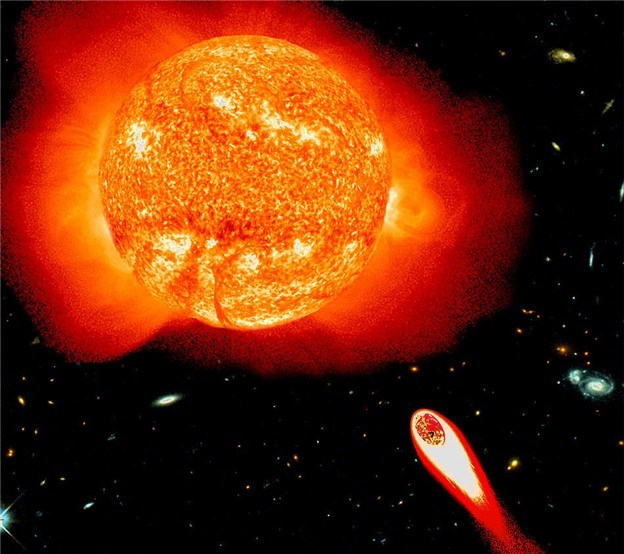# ArmageddonIf the Earth were suddenly stopped in its orbit, how long in days would it take for it to collide with the Sun?

Details and Assumptions

• Regard the Sun and Earth as fixed point masses.

• Mass of the Sun, $M=1.989\times 10^{30} \text{kg}$.

• Mass of Earth, $m=5.972\times 10^{24} \text{kg}$.

• Distance Earth to the Sun, $R=1.496\times 10^{8} \text{km}$.

• The gravitational constant, $G=6.674\times 10^{-11} \text{m}^3\text{kg}^{-1}\text{s}^{-2}$.

×